# Civil Engineering - GATE Exam Questions

### Exercise :: GATE Exam Questions - Section 4

11.

The total length (in km) of the existing National Highways in India is in the range of

 A. 15, 000 to 25, 000 B. 25, 000 to 35, 000 C. 35, 000 to 45, 000 D. 45, 000 to 55, 000

Explanation:

No answer description available for this question. Let us discuss.

12.

A direct runoff hydrograph due to an isolated storm with an effective rainfall of 2 cm was trapezoidal in shape as shown in the below figure. The hydrograph corresponds to a catchment area (in sq. km) of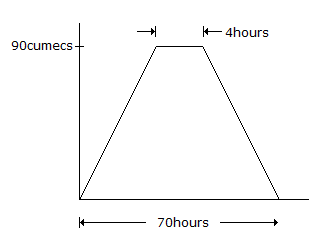A. 790.2 B. 599.4 C. 689.5 D. 435.3

Explanation:

No answer description available for this question. Let us discuss.

13.

A simply supported beam with an overhang is traversed by a unit concentrated moment from the left to the right as shown below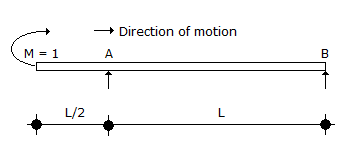The influence line for reaction at B is given by

 A.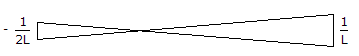B.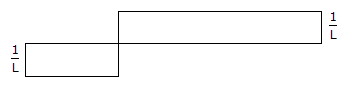C.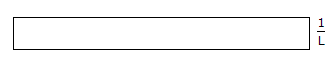D. zero everywhere.

Explanation:

No answer description available for this question. Let us discuss.

14.

The parameters in Horton's infiltration equation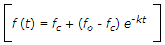are given as, fo = 7.62 cm/hour, fc = 1.34 cm/hour and k = 4.182/hour. For assumed continuous ponding the cumulative infiltration at the end of 2 hours is

 A. 2.68 cm B. 1.50 cm C. 1.34 cm D. 4.18 cm

Explanation:

No answer description available for this question. Let us discuss.

15.

The temporary hardness of water, is caused by:

 A. dissolved carbondioxide B. bicarbonates and carbonates of calcium and magnesium C. bicarbonates of sodium and potassium D. carbonates of calucium and magnesium